# Temperature Dependence

Viscosity is a strong function of temperature. HyperXtrude has five user options for specifying the temperature dependence of polymer viscosity. These options are:

• None
• Exp(Q/RT)
• Exp(Tb/T)
• Exp(-Beta(DeltaT))
• WLF

Besides polymer viscosity, other material parameters can also be specified as a function of temperature using the Function/Table format.

## TemperatureDependence="None"

No additional parameters are required for this option. This option implies that viscosity is not a function of temperature and depends only on the shear rate. This option is chosen if the analysis is isothermal or if the variation of viscosity with temperature is negligibly small.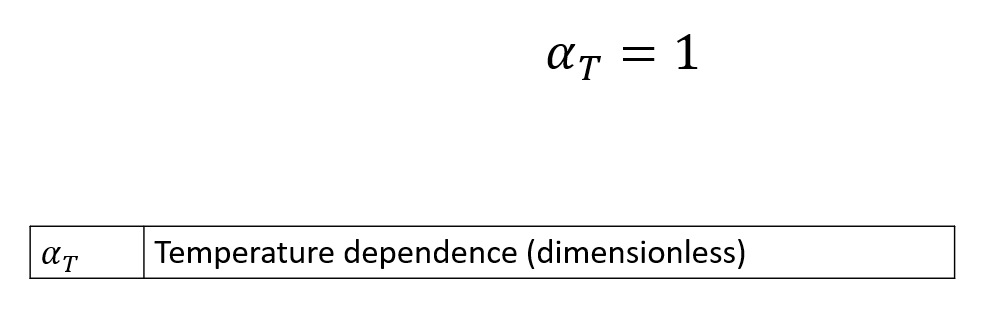Parameter Description Units Data Type Typical Value
TemperatureDependence Specifies one of the five functions available in HyperXtrude for computing temperature dependence of viscosity. None String "None"

## TemperatureDependence="Exp(Q/RT)"

The following equation describes this temperature dependence which is also known as Arrhenius function. R is the universal constant and its value is 8.314 in SI units. The only parameter that needs to be experimentally determined is the activation energy Q. This function is one of the commonly used methods to describe the variation of a property with respect to temperature.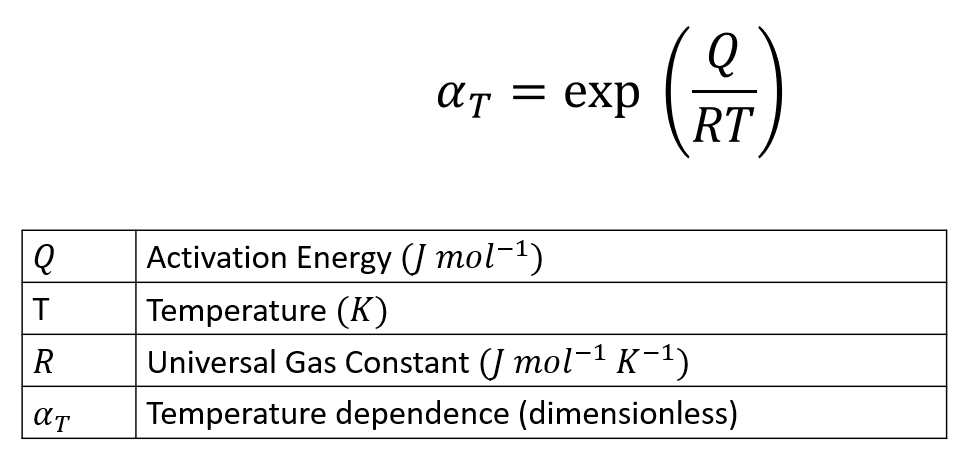The parameters associated with this function are summarized in the following table.

Parameter Description Units Data Type Typical Value
TemperatureDependence Specifies one of the five functions available in HX for computing temperature dependence of viscosity. None String "Exp(Q/RT)"
ReferenceTemperature Temperature at which data is calculated for the initialization step. K Constant 533
FreezeTemperature This is the no flow temperature. Below this temperature material ceases to flow. K Constant 350
ActivationEnergy Experimentally determined parameter to define the temperature sensitivity. J/mol Constant 16628
UniversalGasConstant A parameter from state equation PV = nRT, R is universal Gas constant. J/mol/K Constant 8.314

## TemperatureDependence="WLF"

The William-Landel-Ferry (WLF) equation is often used with semi-crystalline polymers. This function describes the variation in the property with respect to the data at the glass transition temperature (Tg). It is important to note that C1 is a constant and has no units. The parameter C2 is like temperature difference (deltaT) and its value is same in K and deg C.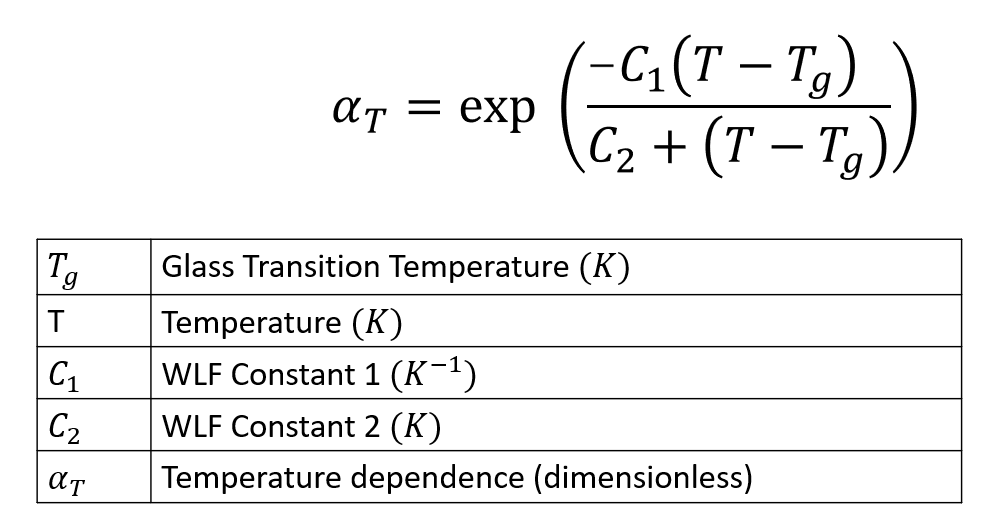Parameter Description Units Data Type Typical Value
TemperatureDependence Specifies one of the five functions available in HX for computing temperature dependence of viscosity. None String "WLF"
ReferenceTemperature Temperature at which data is calculated for the initialization step. K Constant 533
FreezeTemperature This is the no flow temperature. Below this temperature material ceases to flow. K Constant 350
WLFConstant1 Constant C1 of WLF model None Constant 17.44
UWLFConstant2 Constant C2 of WLF model. This is like DeltaT, hence the value is same in K and Celsius. K Constant 51.6
GlassTransitionTemperature Temperature below with polymer molecules ceases to move (frozen). There are few definitions of this term. K Constant 320

## TemperatureDependence="Exp(-Beta(DeltaT))"

This function is mostly used in academic examples and is not as popular as the Arrhenius and the WLF functions. Variation in the viscosity is expressed with reference to the ReferenceTemperature T_0. The parameter beta is experimentally determined.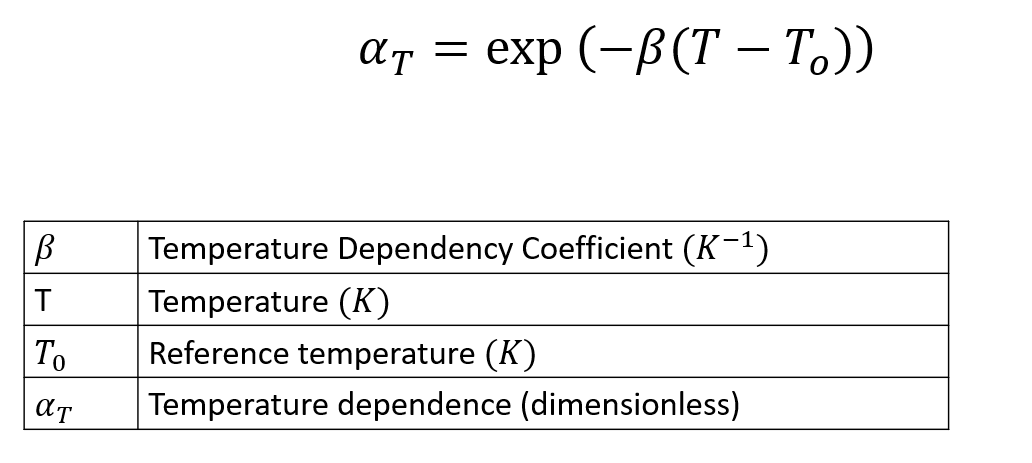Parameter Description Units Data Type Typical Value
TemperatureDependence Specifies one of the five functions available in HX for computing temperature dependence of viscosity. None String "Exp(Tb/T)"
ReferenceTemperature Temperature at which data is calculated for the initialization step. K Constant 533
FreezeTemperature This is the no flow temperature. Below this temperature material ceases to flow. K Constant 350
Beta Parameter in the relationship Exp(-Beta(DeltaT)) None Constant 0.005

## TemperatureDependence="Exp(-Beta(DeltaT))"

This function is mostly used in academic examples and is not as popular as the Arrhenius and the WLF functions. Variation in the viscosity is expressed with reference to the ReferenceTemperature T_0. The parameter beta is experimentally determined.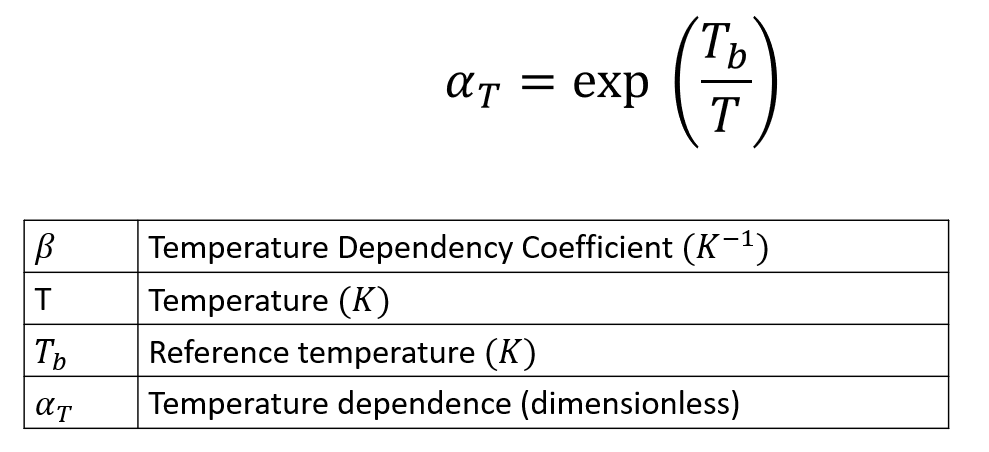Parameter Description Units Data Type Typical Value
TemperatureDependence Specifies one of the five functions available in HX for computing temperature dependence of viscosity. None String "Exp(Tb/T)"
ReferenceTemperature Temperature at which data is calculated for the initialization step. K Constant 533
FreezeTemperature This is the no flow temperature. Below this temperature material ceases to flow. K Constant 350
TemperatureSensitivity A derived parameter which has the same physical meaning as Q/R. K Constant 2000 K